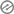# What is the probability of obtaining a multiple of 2 from he cast of a die 🎲

When rolling a fair six-sided die 🎲, there are three outcomes that result in a multiple of 2: 2, 4, and 6. Since there are a total of six equally likely outcomes (numbers 1 through 6) when rolling a standard die, the probability of obtaining a multiple of 2 is the ratio of favorable outcomes to total possible outcomes:

Probability of obtaining a multiple of 2 = (Number of favorable outcomes) / (Total number of possible outcomes) = 3 / 6 = 1/2.

So, the probability of obtaining a multiple of 2 from rolling a fair six-sided die is 1/2 or 0.5.[mediator_tech]

1. When rolling a fair six-sided die 🎲, how many multiples of 2 are possible?
(a) 2
(b) 3
(c) 4

2. What is the probability of getting a multiple of 2 when rolling a die?
(a) 1/6
(b) 1/3
(c) 1/23. Out of the numbers 1 through 6, how many are considered multiples of 2?
(a) 2
(b) 3
(c) 4

4. If a fair six-sided die is rolled, the chance of getting a multiple of 2 is _______.
(a) 1/6
(b) 1/3
(c) 1/2

5. What is the likelihood of rolling a multiple of 2 on a standard six-sided die?
(a) 1/6
(b) 1/3
(c) 1/26. When a fair die is cast, the probability of rolling a multiple of 2 is _______.
(a) 1/6
(b) 1/3
(c) 1/2

7. If you roll a six-sided die, what is the chance of getting a multiple of 2?
(a) 1/6
(b) 1/3
(c) 1/2

8. Among the numbers 1 through 6, how many are even (multiples of 2)?
(a) 2
(b) 3
(c) 49. The probability of rolling a multiple of 2 on a fair six-sided die is _______.
(a) 1/6
(b) 1/3
(c) 1/2

10. What fraction of the outcomes when rolling a standard die are multiples of 2?
(a) 1/6
(b) 1/3
(c) 1/2

11. When rolling a die, the chance of obtaining a multiple of 2 is _______.
(a) 1/6
(b) 1/3
(c) 1/212. If you roll a six-sided die, the likelihood of getting a multiple of 2 is _______.
(a) 1/6
(b) 1/3
(c) 1/2

13. Out of the numbers 1 through 6, how many are divisible by 2 (multiples of 2)?
(a) 2
(b) 3
(c) 4

14. The chance of rolling a multiple of 2 on a standard six-sided die is _______.
(a) 1/6
(b) 1/3
(c) 1/215. If a die is rolled, the probability of obtaining a multiple of 2 is _______.
(a) 1/6
(b) 1/3
(c) 1/2